# Removal of Pb(II) by Modified Natural Adsorbent; Thermodynamics and Kinetics Studies

Document Type : Original Research Paper

Authors

1 Materials and Nuclear Fuel Research School, Nuclear Science and Technology Research Institute, Tehran, Iran

2 Department of Chemical Engineering, Faculty of Engineering, University of Tehran, Tehran, Iran

Abstract

In the current work, the natural zeolite was modified with cobalt hexacyanoferrate and employed for adsorbent of Pb(II) ions from aqueous solution. The modification was approved by XRD and FTIR techniques. The Pb(II) adsorption capacity enhanced by 1.8 times from 60 mg/g (natural zeolite) to 100 mg/g (modified zeolite) at optimal conditions. Factors such as time, pH, temperature, adsorbent dosage and initial concentration were investigated to optimize the adsorption condition. A fast sorption was observed in the initial contact time and equilibrium was achieved in less than 120 min. The optimum pH for lead removal was between 3 and 6. The adsorption capacity was increased and reached the maximum of 90 % at 2 g/L adsorbent dosage. Also, the adsorption increased as the concentration increased up to 500 mg/L and the sorption became constant at higher concentration. It was found that the double-exponential model describes the lead sorption kinetics and the Langmuir–model describe the isotherms.

Keywords

#### Full Text

INTRODUCTION

Water polluted by heavy metals has been considered as a serious problem for their harmful effects on the environment and living organisms, due to their bio-accumulation tendency and persistency in nature. With the rapid development of industries such as battery manufacturing, mining, and smelting factory, wastewaters containing Pb(II) ions are

The aim of the current work was to the treatment of natural zeolite as an adsorbent for Pb(II) removal from aqueous solution. The effects of solution pH, contact time, initial Pb(II) concentration, and adsorbent dosage were investigated.

EXPERIMENTAL

Materials, Characterization, and Modification

All chemicals used in this study including of CoCl2.6H2O, K3Fe(CN)6, and Pb(NO3)2were analytical grade from Merck Co. The Pb(II) stock solutions were prepared by dissolving the lead nitrate salts in deionized water. X-ray diffraction patterns of the natural and modified zeolites were recorded by means of an STOE XD-3A X-ray diffractometer (Cu K𝛼 radiation, k = 0.154 nm, 40 kV and 30 mA). The Infrared spectra of the natural and modified zeolites were recorded by means of a BOMEM MB-series FTIR spectrometer within the wave number range of 250 to 4000 cm-1 after pelleting with KBr. The modification of natural zeolite was performed according to our previous work . 5 g of the dried and powdered zeolite was added to a 100 ml solution of 0.1M Co(NO3)2 at 25 °C for 2 hours. Then the zeolite was washed and mixed with a 100 ml of 0.1M K4Fe (CN)6 solution to deposition of the CoHCF in the surface and channels of zeolite. Then the treated zeolite was washed and dried at 60 °C for 2 hours.

Pb(II) Sorption

The batch adsorption experiments were performed according to the following: a determined amount of the adsorbent (0.1g) was added to 20 ml of Pb(II) solutions. The mixture was stirred for a period of time. Then the samples were filtered and the remained Pb(II) ions in the solution were determined by ICP techniques. The Pb(II) removal capacity was defined as: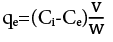(1)

Where v(L) is the volume of Pb(II) solution, Ci (mg/L) is initial Pb(II) concentration, Ce(mg/L) is final Pb(II) concentration, and w(g) is the mass of the solid phase.

The effect of contact time, initial concentration, pH, adsorbent dosage and temperature parameters were studied to determine the modified zeolite properties and the adsorption parameters.

RESULTS AND DISCUSSION

Characterization

The XRD patterns of the natural and modified zeolites are shown in Fig. 1. The characteristic reflection peaks at 2θ= 10°, 11.3°, 13.2°, 22.5°, 27°, 30.02° and 32°, respectively ( belong to clinoptilolite) can be seen in the two samples . In modified zeolite in addition to the clinoptilolite peaks, the characteristic peaks at 2Ѳ = 40◦, 50◦, and 55◦ correspond to (420), (422) and (440) plane of the crystal structure of cobalt hexacyanoferrate also can be seen in pattern (JCPDS card no: 46‐907) which it confirms successful modification of zeolite.

The FT-IR spectra of the natural and modified zeolites are shown in Fig. 1(inset). A sharp peak which appeared at 2090 cm–1, in the modified zeolite is a characteristic of the cyanide group (C≡N) and confirms the successful modification of the natural zeolite [13, 14].

Two main removal mechanisms of Pb(II) by adsorbent are physical adsorption (by negative lattice charge) and ion exchange by cobalt ferrocyanide (K+ by Pb2+) and cations of zeolite. The cobalt ions didn’t contribute in ion exchange. In fact, the high surface area of zeolite and high adsorption affinity of cobalt ferrocyanide lead to synergistic effects of zeolite and metal Hexacyanoferrate and improve selectivity and adsorption capacity towards metal ions.

The effect of temperature on the adsorption of Pb(II) on the modified zeolite was investigated at 298, 313 and 333 K (Fig. 2c, d. Table 1). Temperature change results in changes related to both kinetics and equilibrium of adsorption process. Change in the mass transfer resistance, the thickness of the boundary layer surrounding the adsorbent, surface activity of the adsorbent and kinetic energy of the metal ions are the results of temperature change in the adsorption process. As shown, by the increase of the temperature the adsorption capacity was decreased which indicating that the process was endothermic.

The change in free energy (ΔG), enthalpy (ΔH) and entropy (ΔS) of Pb(II) adsorption were determined from the following equation:

ΔG ͦ =-RT LnK(2)

Where T(K) is temperature, R is gas constant, and Kc is the equilibrium constant. The Kc was determined from the following equation:

Kc= Ce/Cs W/V (3)

Where Ce and Cis the equilibrium concentration of Pb(II) on adsorbent (mg/L) and in the solution (mg/L).

The negative value of ΔH indicating the exothermic nature of Pb(II) adsorption on the modified zeolite. The negative value of ΔS reflects an ordered arrangement of ions around adsorbent particles (at solid/liquid interface) and the negative ΔG indicate the spontaneous nature of the process.

Time Effect

The rate of adsorption is one of the important criteria for the efficiency of adsorbent and also the mechanism of adsorption can be concluded from kinetic studies. Therefore the study of adsorption kinetics is vital in the adsorption process. So for optimize of experimental condition and determine of kinetic parameters, the sorption capacity was measured at different interval contact times (15-480 min, at 298 ͦ C). The result indicated that in the initial contact time equilibrium was achieved (in less than 120 min). At initial contact step, the arge concentration gradient between the solution and adsorbent surface lead to increasing of adsorption rate . Then, the adsorption rate is diminished, because more sorption sites were occupied .

The pseudo-first-order, pseudo-second-order, double-exponential, Elovich, and intraparticle diffusion models were used for the fitting of the experimental data (Fig. 3, Table 2).

The pseudo-first-order rate as earliest and common model for describing the adsorption rate is expressed as:

where qe and qt are the amounts of the ions adsorbed (mg·g−1) on the modified zeolite in equilibrium and at time t, respectively, and K1 (min−1) is the rate of the constant adsorption.

The pseudo-second-order rate model is expressed as: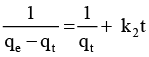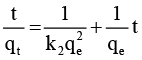where k2 is the rate constant of the pseudo-second-order equation (g/mg min)[9-11].

when the initial adsorption rate h (mg L-1 h) is:

The mathematical equation of intraparticle diffusion kinetic model is as:

Where kad is the rate constant of intraparticle transport (mg/g min1/2), and c is the boundary layer diffusion [9-11].

The Elovich equation which describes the adsorption rate on the adsorbent decreases exponentially with an increase in the amount of the adsorbed material as:

dq/dt = ae-αq

Where q represents the number of ions adsorbed at time t, a desorption constant, and α the initial adsorption rate [9-11].

Chemical reaction and diffusion steps determine the total rate of the adsorption process. Diffusion could have steps such as transfer in solution or in solid. The correlation coefficients were used for the evaluation of the compatibility of experimental data with models. The R2 of the double-exponential model is the highest value compared with other models. So the double-exponential model can describe the data better than other models. In the double-exponential model, the adsorption of ions by adsorbent occurs in two steps. A rapid first step involving external and internal diffusions, followed by a slow step involving the intraparticle diffusion. The double-exponential model is expressed by the following Equation [18, 19]:

where Dhas rapidly adsorbed fractions of ion (mg/l), D2 is the amounts of slowly adsorbed fractions of ion (mg/l), and KD1 and KD2 are rapid and slow rate constants (min–1) respectively. The model parameters of the slow and rapid steps extracted from the experimental data are presented in Table 2.

Isotherms

The effect of initial concentration on the adsorption capacity was studied by changing Pb(II) initial concentrations at the range of 10–1500 mg/L. The results indicated that the adsorption increased as the concentration increased up to 500 mg/L and the sorption became constant at higher concentration. The obtained data were fitted with The Langmuir, Freundlich, Dubinin–Radushkevich, and Temkin isotherm models (Fig. 4). The extracted isotherm parameters, the correlation coefficients (R2) are listed in Table 3. The correlation coefficients (R2) of isotherm equations for Pb(II) was much closer to unity for the Langmuir model than another model. The nonlinear form of Langmuir isotherm can be expressed as[11-17]: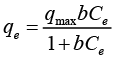(5)

Where qe (mg/g) is the amount adsorbed per unit mass of adsorbent at equilibrium concentration; C(mg/L) is the equilibrium concentration of the adsorbate; qmax (mg/g) is the maximum adsorption capacity; b is the adsorption equilibrium constant, characteristic of the affinity between the adsorbent and adsorbate [11-17].

The non-ideal and reversible adsorption in multilayer would be described by Freundlich isotherm, and the linear form of the equation is: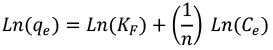where C(mg L-1) is the equilibrium concentration of ions, qe (mg g-1) the amount of adsorbed ions, n and KF (mg/g) are Freundlich constants related to the intensity of the adsorption and the sorption capacity respectively. In the Temkin isotherm, the interactions between the sorbed species are considered and enthalpy of all the adsorbed molecules in solution is accomplished. The Temkin isotherm is as follows:

qe = BLnA+ BLnCe

Where B (J/mol) and AT (L/g) are the constants related to the sorption heat and Temkin isotherm equilibrium binding respectively. From the slope and intercept of the qe vs. lnCe curve, the constants could be determined.

The Dubinin-Radushkevich (D-R) isotherm is as the following:

Lnqe= Lnqm - BDR ξ2

Where, qm is the maximum of adsorbed ion by adsorbent (mmol/kg), BDR is a constant related to the sorption energy (mol2/kJ2); and ε is the Polanyi potential (RT ln (1 + 1/Ce)), where R and T are the gas constant (kJ/mol.K), the absolute temperature respectively. The ε is equal to: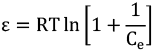Where Ce is the adsorbate equilibrium concentration (mg/lit) .

CONCLUSION

The cobalt ferrocyanide modified zeolite was applied for removal of Pb(II) from aqueous media. The Pb(II) adsorption capacity was increased from 60 to 100 mg/g by modification of natural zeolite. The equilibrium was achieved in less than 120 min. The optimum pH was between 3 and 6. The adsorption capacity was increased and reached the maximum of 90 % at 2 g/L adsorbent dosage. The adsorption increased as the concentration increased up to 500 mg/L and the sorption.

CONFLICTS OF INTEREST

The authors declare that there are no conflicts of interest.

#### References

REFERENCES
1. H.H.A. Ghafar, A.M. Abdel-Aty, N.S. Ammar, M.A. Embaby, Lead biosorption from aqueous solution by raw and chemically modified green fresh water algae
Scenedesmus obliquus, Desalin. Water Treatو. 52 (2014) 7906-7912.
6. M.E. Ulmanu, E. Maran, Y. Fernndez, L. Castriln, I. Anger, D. Dumitriu, Removal of Copper and Cadmium Ions from Diluted Aqueous Solutions by Low Cost and Waste Material Adsorbents, Water Air Soil Pollut., 142 (1–4) (2003) 357–373.
11. T. Yousefi, H. R. Moazami, H. R. Mahmudian, M. Torab-Mostaedi, M. A. Moosavian, Modification of natural zeolite for effective removal of Cd(II) from wastewater, J. Water Environ. Nanotechnol., 3(2) (2018) 150-156.
14. M. Kazemimoghadam, Z. Amiri Rigi, Evaluation and synthesis of Nano-pore Hydroxysodalite (HS) zeolite membranes: Application to pervaporation of Ethanol/water mixture, J. Water Environ. Nanotechnol., 3(2) (2018) 173-190.
16. M. Moses,M. Mbugua Margaret, M. Nga’ng’a Benson Wachira Harun. Mbuvi, Removal of Lead Ions and Turbidity from Waste Water by Adsorbent Materials Derived from Cactus Leaves, J. Nat. Sci. Res., 6 (2016) 63-72.